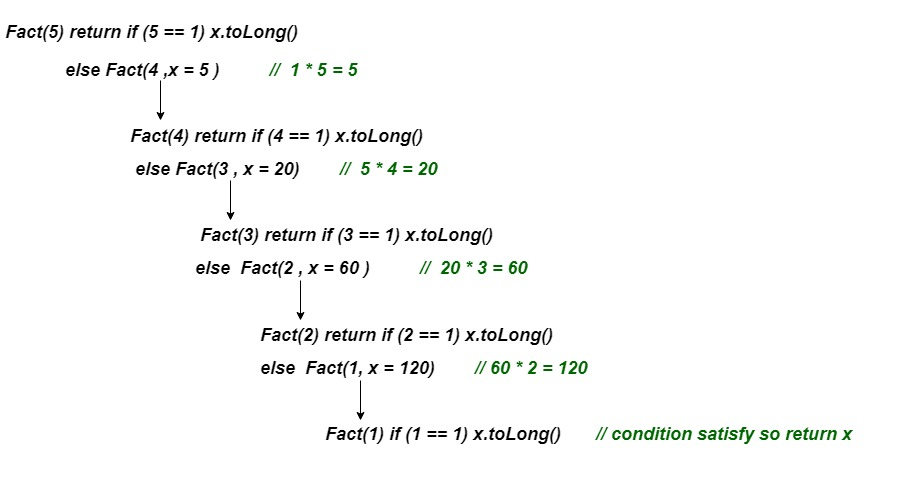Open in App
Not now

# Kotlin Tail Recursion

• Difficulty Level : Medium
• Last Updated : 28 Mar, 2022

In traditional recursion call, we perform our recursive call first, and then we take the return value of the recursive call and calculate the result. But in tail recursion, we perform the calculation first, and then we execute the recursive call, passing the results of the current step to the next recursive call. In the end, both recursion and tail recursion gives same output. The must follow rule for the tail recursion is that recursive call should be the last call of the method.

### Benefits of using tail recursion –

1. In tail recursion, function call is the last thing executed by the function and nothing left in the current function to execute. So, there is no need to save current function call in stack memory and compiler can re-use that stack space for next recursive call.
2. In tail recursion, we do not get the StackOverflowError during the execution of program.

Example 1: Find the factorial of a number using tail-recursion.

## Kotlin

 `// Kotlin program of factorial using tail-recursion` `fun Fact(num: Int, x:Int):Long{`   `    ``return` `if``(num==``1``)   ``// terminate condition` `        ``x.toLong()` `    ``else` `        ``Fact(num-``1``,x*num)   ``//tail recursion` `}` `fun main() {` `    ``var n = ``1` `    ``var result = Fact(``5``,n)` `    ``println("Factorial of ``5` `is: \$result")` `}`

Output:

`Factorial of 5 is: 120`

Working of the above program-Example 2: Find the sum of elements of an array using tail-recursion

## Kotlin

 `// two parameters passed an array and size of array` `fun sum(args: Array , index:Int, s : Int = ``0` `):Int{` `    ``return` `if``(index<=``0``) s` `    ``else` `sum(args ,index-``1``, s + args[index-``1``])     ``// tail-recursion` `}`   `fun main() {` `    ``// array initialization` `    ``val array = arrayOf(``1``,``2``,``3``,``4``,``5``,``6``,``7``,``8``,``9``,``10``)` `    ``// size of array` `    ``val n = array.size` `    ``val result = sum(array,n)             ``// normal function call` `    ``println("The sum of array elements is: \$result")` `}`

Output:

`The sum of array elements is: 55`

Explanation: Here, we have passed the array as an argument along with two other parameters in sum() function. The default value of (s) parameter is equal to zero. We are calculating the sum of elements, from the last index of the array, with each recursive call. With the last recursive call, we will have the sum of all elements in s and return it when condition satisfies.

My Personal Notes arrow_drop_up
Related Articles